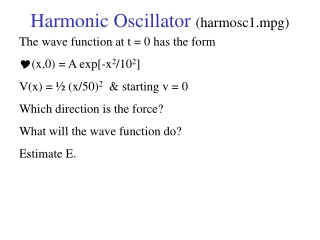DownloadDownload PresentationHarmonic Oscillator (harmosc1.mpg)

# Harmonic Oscillator (harmosc1.mpg)

Download Presentation## Harmonic Oscillator (harmosc1.mpg)

- - - - - - - - - - - - - - - - - - - - - - - - - - - E N D - - - - - - - - - - - - - - - - - - - - - - - - - - -
##### Presentation Transcript

1. Harmonic Oscillator (harmosc1.mpg) The wave function at t = 0 has the form Y(x,0) = A exp[-x2/102] V(x) = ½ (x/50)2 & starting v = 0 Which direction is the force? What will the wave function do? Estimate E.

2. Harmonic Oscillator (harmosc1.mpg)

3. Harmonic Oscillator (harmosc1.mpg) Why doesn’t |Y|2 change with t? Does Y change with t? If used Y(x,0) = A exp[-x2/112], would |Y|2 change with t?

4. Harmonic Oscillator (harmoscr.mpg) The wave function at t = 0 has the form Y(x,0) = A exp[-x2/102] V(x) = ½ (x/50)2 & starting v = 0 Only the real part of Y in this movie

5. Harmonic Oscillator (harmoscr.mpg)

6. Harmonic Oscillator (harmoscr.mpg) What is the real part of Y? The imaginary part? Why is the solution a function of x times a function of t?